Next: Conclusions Up: Evolution of the Double Previous: Calculation of Binary Neutron

## Effect of sources evolution on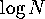-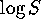distribution and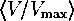-test

We conventionally assume a flat Universe so that, withandbeing fractional contributions of matter and cosmological constant term (Carroll et al., 1992). The present value of the Hubble constant is assumed to be 75ĀkmĀsMpc. The GRB were considered as standard candles with a proper luminosity L and have a power-law spectrum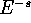with a spectral index s=1.5 (Schaefer et al., 1992). Briefly, the count rate at the detector from a source at a redshift z is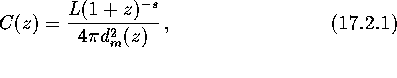whereis a metric distance (see Carroll et al., 1992). The number of events, N(>C), with an observed count rate exceeding C is thus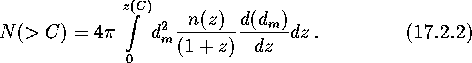The source evolution n(z) can easily be obtained using the evolutionary Green function: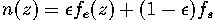and the dependence t(z) for a particular cosmological model.

Figure 55 shows the ``Green functions'' for the double compact binary merging rateĀ evolution assuming no collapse anisotropy.Ā A very strong early evolution with time is seen; however, even for an elliptical galaxyĀ the NS+NS merging rate is 1 peryr for an age ofyr. The non-monotonic character of the merging rate evolution is due to different contributions of different type binaries (by initial masses, separations and types of first mass exchange); as the detailed shape is of less importance for us now, we postpone discussing these interesting features to a separate paper. We should, however, note that a small decrease in the coalescenceĀ rate observed at the age of about 4 billion years is statistically significant and is caused by contributions of evolutionary different types of NS+NS binaries (they originate from different ranges of initial mass ratios and semi-major axes). This feature can produce a notable decrease in the-curve slope at the corresponding. Thus, ifthe drop in sources production rate after 4 billion years from the beginning corresponds (for the flat Universe), somewhat surprisingly, just to the feature visually seen in both second and third BATSEĀ catalog-curves; however, it would be prematurely to take the apparent feature seriously due to its low statistical significance. Another way that diverse peculiarities in the-distribution induced by the sources evolution could arise is connected with a possible non-monotonic character of the star formation rate in galaxies.

ĀFigure 55: Temporal evolution of NS+NS (filled circles) and NS+BH (open circles) coalescenceĀ rates calculated forbinaries and normalized to a model elliptical galaxy with baryonic massĀĀ (Lipunov et al., 1995e).

For aspiral galaxyĀ with constant star formation rate our calculations give a binary NS coalescenceĀ rate of the order of 1 per 5000Āyr only slightly depending on the initial mass ratio spectrum and assumptions about massive core collapse anisotropy.Ā This rate is close to the most ``optimistic'' estimates based on evolutionary considerations (Lipunov et al., 1987a, 1995a,e; Tutukov and Yungelson, 1993a; van den Heuvel, 1994). In our calculations, we used a smoothed evolutionary function assuming the star formation to occur during the first 500ĀMyr in elliptical galaxies,Ā and constant in spiral galaxies.Ā In fact, the results proved to be only weakly sensitive to the smoothing over time provided that it is less than 1 billlion years (the width of the wide peak of the Green function; see Figure 55).

Our theoretical models depend on three unknown parameters,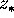,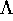,, as well as on s. The calculated-distributions were compared with the BATSEĀ data by using the Mises-Smirnovtest (which gives essentially the same results as the Kolmogorov-Smirnov test, but uses a more smooth criterion for comparing observed and tested distribution functions; see Bol'shev and Smirnov (1965)). FigureĀ56 shows two-dimensional cuts through the parameter space. All three cuts show contour lines for confidence level according totest higher than 90 percent, with the maximum level being at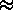95 percent in all planes.

ĀFigure 56: Confidence level contour lines (90 percent, 91 percent, ...) fortest in theplane at(a), in the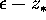plane for(b) and in theplane for(c). A flat cosmological model and GRB spectral index s=1.5 with the source evolution as in FigureĀ55 is adopted.Ā

The calculated-distributions are plotted in FigureĀ57 for different star formation starting times. Other parameters are fixed at the best-fit valuesand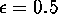. The most prominent feature of the theoretical-obtained is a notable sharp increase at low count rates due to evolutionary effects. In terms of-test, such a turn-up of the-curve would lead to a sharp increase of the averagevalues when weaker sources (i.e. farther sample limits) are taken into account (FigureĀ58). The curves turn out to be most sensitive to the parameterand depend only slightly on other parameters. We also note that a flatter GRB spectral index s=1 would favour earlier initial star formationand changes the-curves more at lower.

ĀFigure 57:-diagram simulated for sources evolving as shown in FigureĀ55 in a flat cosmological model with the best-fit vacuum energyand parameter.Ā (Lipunov et al., 1995e).

Ā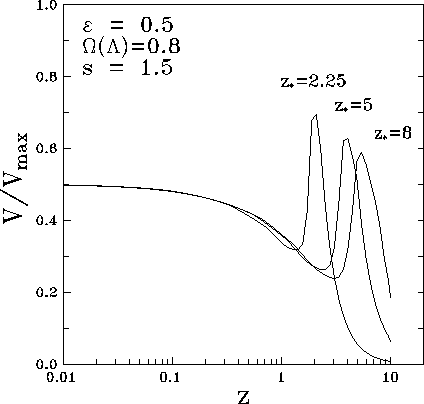Figure 58: Dependence of the differential-test on sample limit redshift z for different epochs of the primordial star formationĀ (Lipunov et al., 1995e).

If cosmological binary NS coalescencesĀ does underly the GRB phenomenon, late epochs of initial star formation in galaxies () would be inconsistent with the already existing BATSEĀ data.Next: Conclusions Up: Evolution of the Double Previous: Calculation of Binary Neutron

Mike E. Prokhorov
Sat Feb 22 18:38:13 MSK 1997